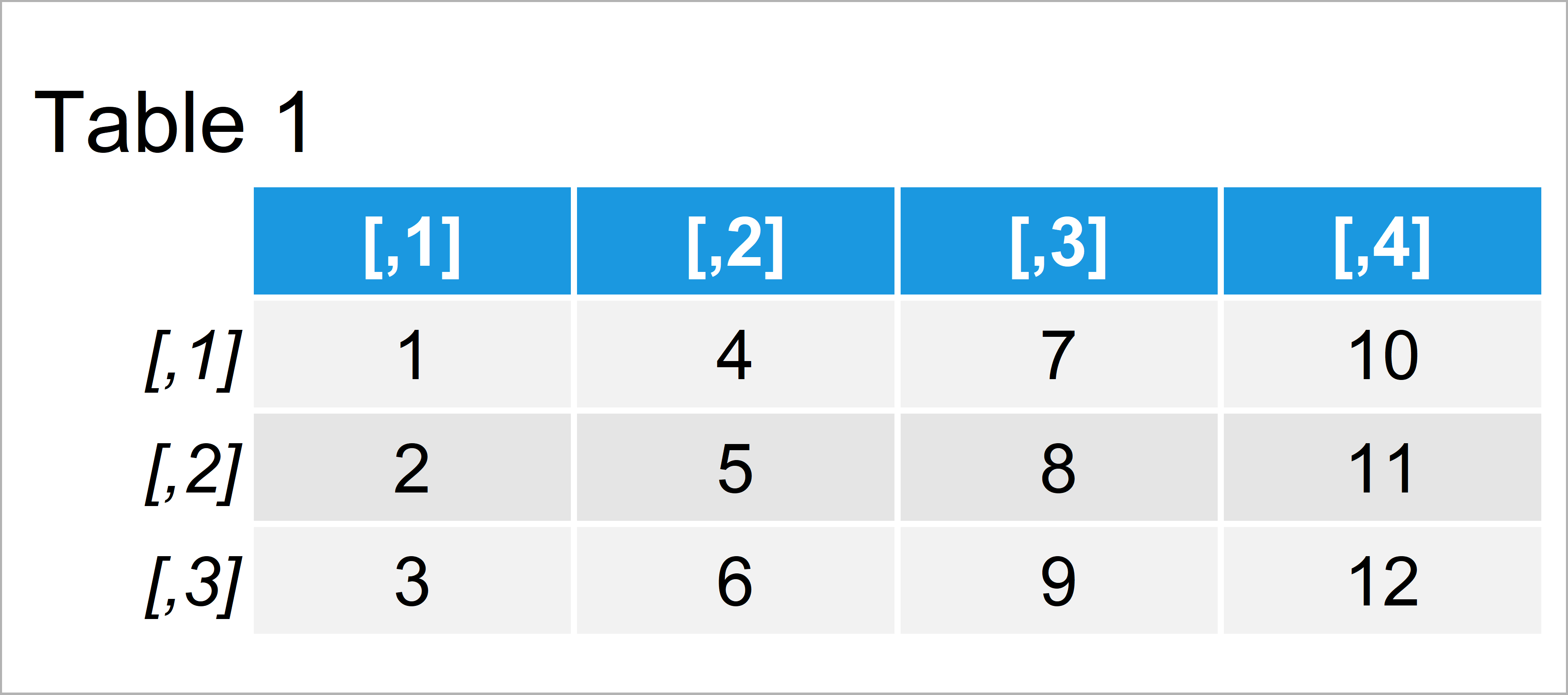# Convert Character Matrix to Numeric in R (Example)

In this tutorial, I’ll show how to set all columns of a character matrix to numeric in R programming.

The table of content looks as follows:

Let’s do this!

## Constructing Example Data

As the first step, let’s construct some example data:

```mat_char <- matrix(as.character(1:12),     # Create character matrix
ncol = 4)
mat_char                                   # Print character matrix```Table 1 shows the structure of our example data – It has three rows and four columns. All elements of our example matrix have the character class.

## Example: Convert Character Matrix to Numeric Matrix Using as.numeric() & matrix() Functions

The following code explains how to convert an entire matrix from the character string data type to the numeric class in R.

The basement of our conversion is the as.numeric function. If we apply the as.numeric function to our matrix, we get the following vector output:

```as.numeric(mat_char)                       # Convert to numeric vector
#    1  2  3  4  5  6  7  8  9 10 11 12```

We can combine the as.numeric function with the matrix function and the ncol argument to convert our matrix to the numeric class:

```mat_num <- matrix(as.numeric(mat_char),    # Convert to numeric matrix
ncol = ncol(mat_char))
mat_num                                    # Print numeric matrix```As shown in Table 2, the previously shown R programming code has created a numeric matrix in R.

## Video, Further Resources & Summary

Please accept YouTube cookies to play this video. By accepting you will be accessing content from YouTube, a service provided by an external third party.If you accept this notice, your choice will be saved and the page will refresh.

In addition, you may read the related R tutorials of this website. You can find a selection of posts here:

Summary: In this article you have learned how to convert a character matrix to the numeric class in R. If you have additional questions, let me know in the comments.

Subscribe to the Statistics Globe Newsletter